## Central Limit Theorem

Letbe a set of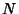Independent random variates and eachhave an arbitrary probability distribution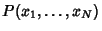with Mean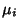and a finite Variance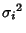. Then the normal form variate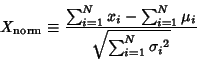(1)

has a limiting distribution which is Normal (Gaussian) with Meanand Variance. If conversion to normal form is not performed, then the variate(2)

is Normally Distributed withand. To prove this, consider the Inverse Fourier Transform of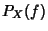.(3)

Now write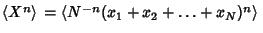(4)
so we have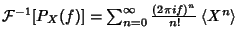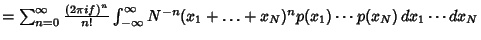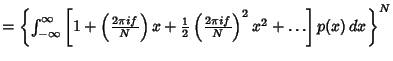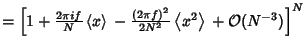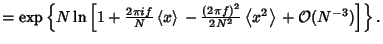(5)
Now expand(6)

so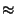(7)

since(8)(9)

Taking the Fourier Transform,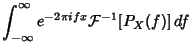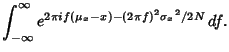(10)

This is of the form(11)

whereand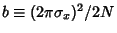. But, from Abramowitz and Stegun (1972, p. 302, equation 7.4.6),(12)

Therefore,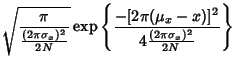(13)

Butand, so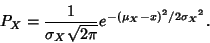(14)

The fuzzy'' central limit theorem says that data which are influenced by many small and unrelated random effects are approximately Normally Distributed.

References

Abramowitz, M. and Stegun, C. A. (Eds.). Handbook of Mathematical Functions with Formulas, Graphs, and Mathematical Tables, 9th printing. New York: Dover, 1972.

Spiegel, M. R. Theory and Problems of Probability and Statistics. New York: McGraw-Hill, pp. 112-113, 1992.

Zabell, S. L. Alan Turing and the Central Limit Theorem.'' Amer. Math. Monthly 102, 483-494, 1995.